Next: Relations with other numerical Up: A remark on quantum Previous: Quantum dynamics versus random

# Numerical experiments

We illustrate the discrete time evolution (2.1) in two numerical experiments.

(i) The first numerical experiment deals with an electron in the plane under a constant magnetic field B reduced by a Landau gauge to a one-dimensional situation, where one considers the one-dimensional Schrödinger operator L on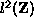of the form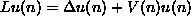and take an initial condition which is a localized wave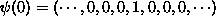at the origin k=0. We use Wiener's theorem to get numerically information about the discrete part of the spectral measure. For this illustration, we take the almost Mathieu (or Harper) operator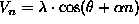, where much about the spectrum is known (see [24, 20, 11] for reviews). Note that most of the known results hold only for almost all or genericand under some assumptions on the magnetic flux.

We did a numerical determination of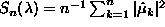using (2.1), up to n=40'000 as a function of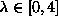in the almost Mathieu operator with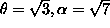.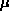is the spectral measure on the circle belonging to the vector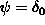localized at the origin in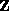. The value of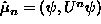was computed using evolution (2.1) with initial condition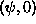on the grid [-n/2,n/2] so that the boundary effects the value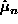only after n steps: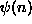has support in [-n,n] and the boundary begins to affect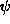after n/2 time steps and so to influenceafter n steps. The numerical experiment is in agreement with the now established fact that there is no point spectrum for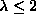for almost all(for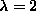see ) and some point spectrum for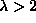. Longer runs, () indicated that indeed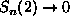for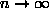.

(ii) In a second numerical experiment, we take a two dimensional operator L which is the Hamiltonian for an electron in the discrete plane, where the magnetic field B is randomly taking values in U(1) (see  for some theoretical results or [3, 2] for other numerical experiments on this model). If the distribution of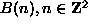is a Haar measure of U(1), then this field can be generated with a vector potentialwith independent random variables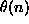having the uniform distribution in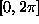. There is no free parameter. The ergodic operator, which we consider, is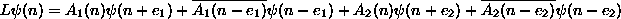and the open question is what is the spectral type of L.

While one knows the moments of the density of states of L (the n-th moment of the density of states is the number of closed paths in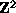of length n starting at 0 which give zero winding number to all plaquettes ), nothing about the spectral type of L seems to be known. For the two dimensional experiment, we experimented on alattice, were we can compute the first 1000 Fourier coefficients of the spectral measure exactly. Our experiments indicate no eigenvalues. If eigenvalues exist, they would have to be extremely uniformly distributed because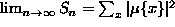must be small. The measurements done on a usual workstation indicate that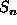goes to zero monotonically: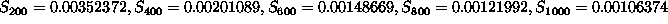. Longer runs with better computers on larger lattices are needed to confirm this picture.

Remark. We made also numerical experiments with a Aharonov-Bohm problem on the lattice. This is the situation when the magnetic field B is different from 1 only at one plaquette n=(0,0). The vector potential A in this situation can not be chosen differently from 1 in a compact set. However, in a suitable gauge, the operator L is a compact perturbation of the free operator by a result of Mandelstham-Jitomirskaja  see  for an other proof of this fact). As expected, there was no indication of some discrete spectrum. The numerical experiments suggest thatis bounded which would mean that the spectral measures are in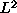.Next: Relations with other numerical Up: A remark on quantum Previous: Quantum dynamics versus random

Oliver Knill, Tue Aug 18, 1998